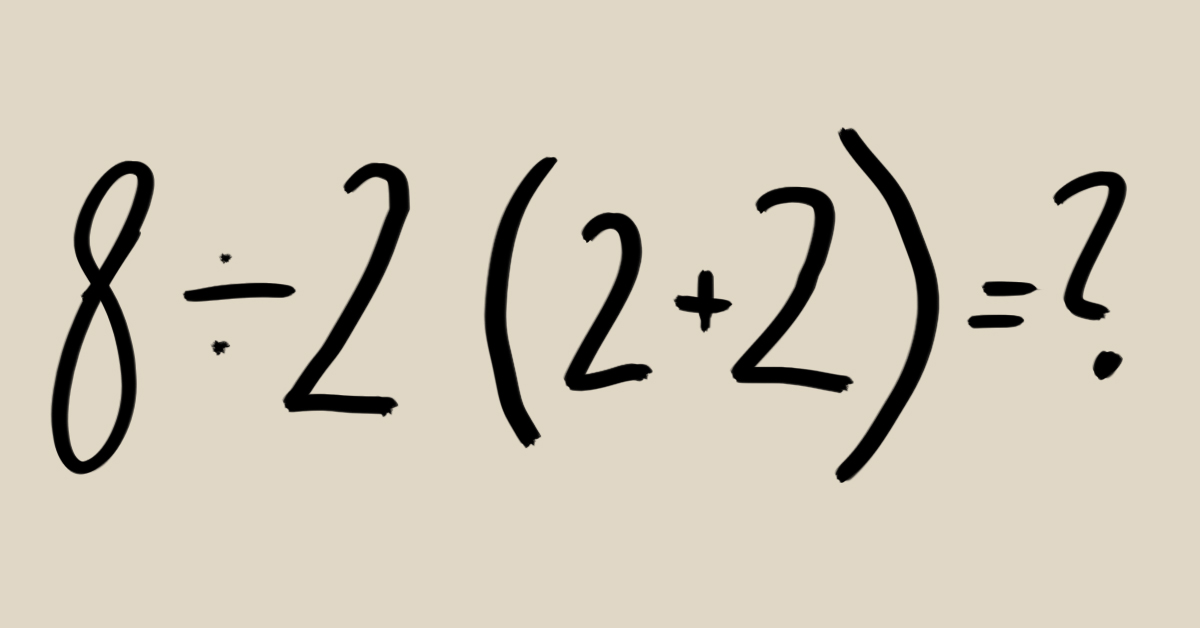# Here’s the Correct Answer to this Viral Math Problem That is Dividing the InternetWe all like to fancy ourselves secret geniuses. We can’t pass up the opportunity to show how smart we are when presented with a problem that has others stumped. But what happens when no one can agree on what the right answer is?

Remember the math problem 48÷2(9+3) and all the arguments it generated? Well, a similar equation has the Internet divided in a feud, and once again Twitter users can’t seem to agree on the answer.

The problem, which was shared by Twitter user @pjmdoll, has divided internet users with some insisting the answer to the equation is 16 while others have 1 as their answer. Below are some of the arguments from both ends of the divide.

The primary conflict seems to be whether to apply the principles of PEMDAS or not. PEMDAS is an acronym used to signify the order for calculating the most important mathematical functions which include Parenthesis, Exponents, Multiplication, Division, Addition, and Subtraction.

When given a math problem which has two or more possible operations at any given time, like with ‘8÷2(2+2),’ PEMDAS tells you the order of what to calculate first, second and so on until you arrive at an answer.

This theory was explained by one user who had this to say:

I have taught math for almost 30 years; the answer is 16. After the 2+2 is resolved to 4, then the problem becomes 8÷2(4), which is the same as 8÷2×4. Since division is on the left, it must be done first. So, 4×4=16. If it were written as 8 OVER 2(4), the answer would be 1.

— Eric Stephen Emmons (@EricStephenEmm2)

However, not everyone agrees with this line of reasoning, as some Twitter users pointed out that the principles of BODMAS could also be used to solve the problem. BODMAS, which is an acronym for Bracket, Of, Division, Multiplication, Addition, and Subtraction, is a synonym of PEMDAS but the difference lies in the way they are both applied.

This user explains how it works:

Answer is 1. (Enough explanations below)

For those who think it is 16 (including math teachers):

8/2(4)=8/8=1

Application of BODMAS means removing operators. You should remove brackets first before moving to the next operator.”
— Vikas (@gvikas)

Here is another insight that simplify things better:

Normally it would be 16 using order of operations properly. Some people consider implicit multiplication (the brackets) take precedence over division and then the answer would be 1. Both are correct depending on how you math.

— No Direction Studios (@NoDirectionCrew)

### Here’s a particular favorite explanation of ours from Twitter user @Erin_change4eve  which summarizes the answer succinctly:

There are two answers because it depends on how you learned math

8÷(2(2+2)) is 1

BUT

8÷2(2+2) is 16

I learned pemdas so 16

But bodmas is 1

I never learned bodmas so I don’t know it exactly, but the equation uses pemdas. People are adding brackets that aren’t already there”

— Erin Kelemen (@Erin_change4eve)

It seems that this confusion is not reserved for just humans as calculators can’t seem to make up their minds, as one user noted.

Some calculators are giving the answer as 1. It’s not just people — even the machines are confused.

### Here’s what the experts have to say

The reason there is so much debate over this math problem is because of the confusing way it’s written. It’s intentionally ambiguous and could be solved either way, depending on if you’re using BODMAS or PEMDAS.

Ferndale High School math teacher, Mr. Kelly insists the answer the equation is 16. According to him, those who got the number 1 as the answer were using an outdated version of the order of operations. Since we are taught to remove the brackets first, we automatically forget to divide before we multiply.

“When it’s multiplication or division, you move left to right, not right to left. So that’s why some students are confused and multiply before divide. The multiplication does not come first in this situation. The division comes first,” says Mr. Kelly.

### To solve this problem;

Firstly divide 8 by 2 = 4

Next, multiply 4 with the sum of (2+2) = 4(4)

Note that you are to move left to right instead of right to left when dividing or multiplying.

#### References:

1. Math problem has Internet divided in feud: Here’s the correct answer“, Mlive. August 2019.
2. This equation has 2 wildly different answers depending on what you learned in school, and it’s dividing the internet“, Insider. July 2019.
3. Oomfies solve this“, Twitter. July 2019.
4. Can you solve this math equation?Twitter. August 2019.
#Health #Mind